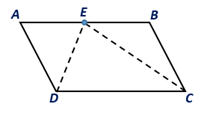Chapter 4.1, Problem 36EElementary Geometry For College St...

7th Edition
Alexander + 2 others
ISBN: 9781337614085

Solutions

Chapter
SectionElementary Geometry For College St...

7th Edition
Alexander + 2 others
ISBN: 9781337614085
Textbook Problem

When the bisectors of two consecutive angles of aparallelogram meet at a point on the remaining side,what type of triangle is: a) ∆DEC? b) ∆ADE? c) ∆BCE?To determine

a.

To find:

The type of the angle in ∆DECwhen the bisectors of two consecutive angles of the parallelogram ABCD meet at a point on the remaining side.

Explanation

Given:

The bisectors of two consecutive angles of the parallelogram ABCD are shown below.

Corollary:

A parallelogram is a quadrilateral in which both pairs of opposite sides are parallel.

In the given parallelogram ABCD.

 PROOF Statements Reasons 1. Parallelogram ABCD 1. Given 2. AD¯∥BC¯ 2. By the definition of a parallelogram 3. ∠EDC=∠D2 and∠DCE=∠C2 3. Since DE and CE are angle bisectors of ∠D and ∠C 4
To determine

b.

To find:

The type of the angle in ∆ADEwhen the bisectors of two consecutive angles of the parallelogram ABCD meet at a point on the remaining side.

To determine

c.

To find:

The type of the angle in ∆BCEwhen the bisectors of two consecutive angles of the parallelogram ABCD meet at a point on the remaining side.

Still sussing out bartleby?

Check out a sample textbook solution.

See a sample solution

The Solution to Your Study Problems

Bartleby provides explanations to thousands of textbook problems written by our experts, many with advanced degrees!

Get Started

Change 4 ft to inches.

Elementary Technical Mathematics

Draw a polygon for the distribution of scores shown in the following table. X f 6 2 5 5 4 3 3 2 2 1

Essentials of Statistics for The Behavioral Sciences (MindTap Course List)

In Exercises 1728, use the logarithm identities to obtain the missing quantity.

Finite Mathematics and Applied Calculus (MindTap Course List)

Using for |x| < 1 and differentiation, find a power series for .

Study Guide for Stewart's Single Variable Calculus: Early Transcendentals, 8th